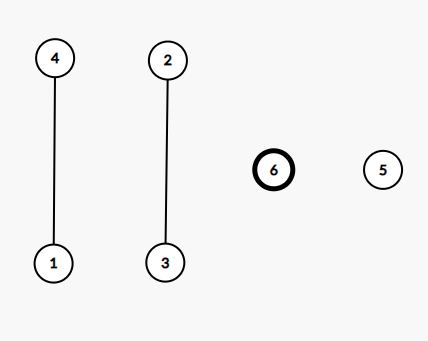# An Interesting Graph Problem - Need help in finding Solution

Given an arbitrary graph , partition the graph into a set of single edges and vertices such that every vertex appears exactly once and the total number of edges and vertices in the set is minimum , i.e the Size of the set should be minimum.

Update 1 :-

let’s take the graph as a simple undirected graph although i don’t think this matters since i think we can treat multigraph and simple graph in the same manner for this particular problem.

Update 2 :-

Every vertex should appear in the partition either as a separate vertex or in a edge but a edge may or may not appear in the partition

For example :-The above graph , can be partitioned into two edges and two vertices and this is one of the minimum size set , this partition size is 4.If anyone have any idea or know any algorithm to solve this problem , please do share it.

Extra info

I encountered this problem while trying to solve the problem PAIRFLIP in the recent long challenge
PAIRFLIP - Editorial - #6 by infinite_king

Final Update :-

I did not find any solution to this problem , if i find any approach in future, i will post the answer here.

What are we exactly trying to minimize ??

Let’s take partition of a graph as a set of single disjoint edges and single vertices such that every vertex appears exactly once and we have to find a partition with the minimum size.

NOTE :- every vertex should appear in the partition either as a separate vertex or in a edge but a edge may or may not appear in the partition

like for the above graph two possible partitions are

P1 = \{ edge(1,4) , edge(2,3) , vertex(6) , vertex(5) \} , the size is 4
P2 = \{ edge(1,2) , vertex(3) , vertex(4) , vertex(6) , vertex(5) \} , the size is 5

For the source ,

I encountered this problem while trying to solve the problem PAIRFLIP in the recent long challenge

problem link :- PAIRFLIP Problem - CodeChef
It editorial :- PAIRFLIP - Editorial

My post about how i connected these two problems
PAIRFLIP - Editorial - #6 by infinite_king